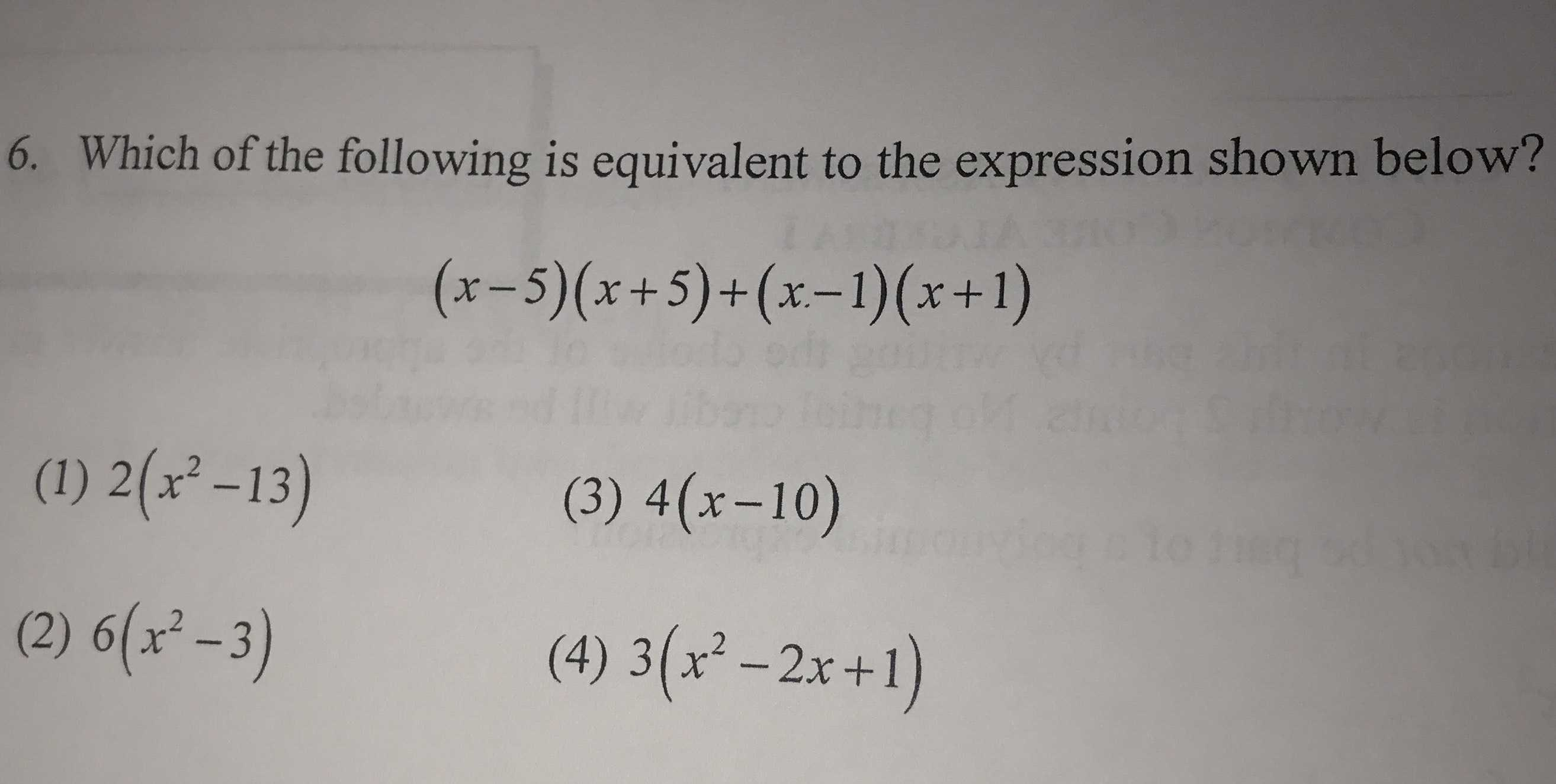### ¿Todavía tienes preguntas de matemáticas?

Pregunte a nuestros tutores expertos
Algebra
Pregunta6. Which of the following is equivalent to the expression shown below?

$$( x - 5 ) ( x + 5 ) + ( x - 1 ) ( x + 1 )$$

(1) $$2 ( x ^ { 2 } - 13 )$$ (3) $$4 ( x - 10 )$$

(2) $$6 ( x ^ { 2 } - 3 )$$ (4) $$3 ( x ^ { 2 } - 2 x + 1 )$$